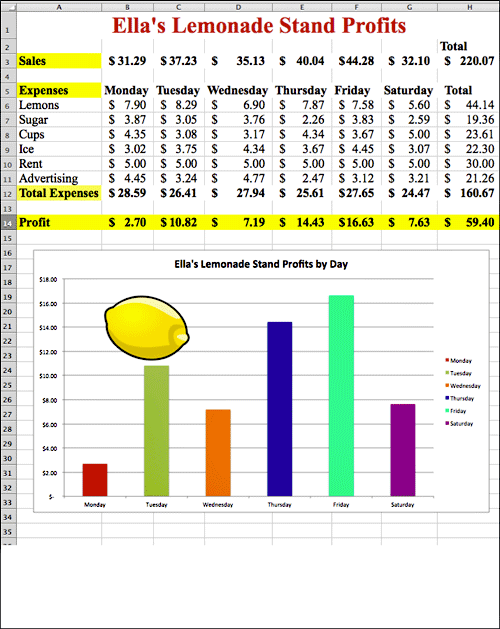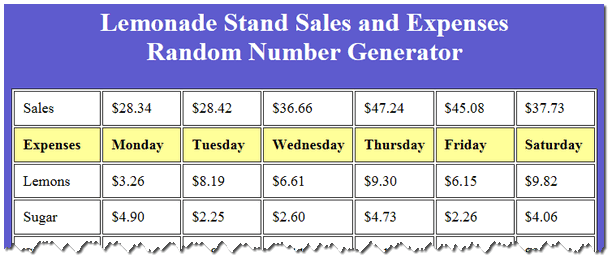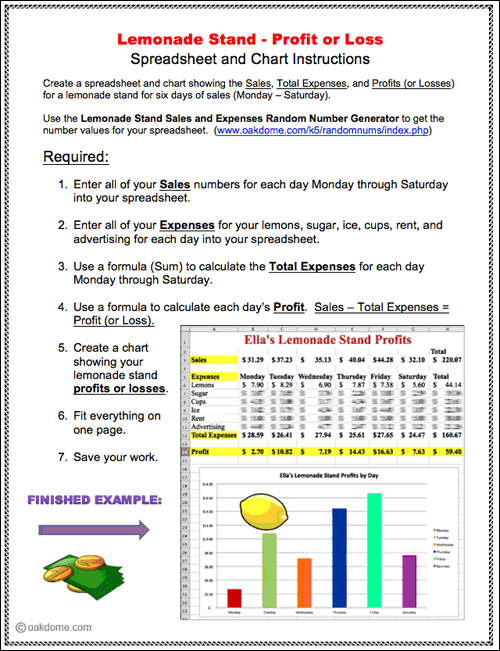Students create a profit or loss spreadsheet and chart for a lemonade stand with 6 days of sales and expenses.

Students use the random number generator to get their sales and expenses numbers for each day.

Students enter their data in the spreadsheet for each day. Students use a formula (sum) to calculate expenses. Students use a simple formula to calculate profits or losses (sales - total expenses = profit ). Grades 4 +

## Lemonade Stand Profit or Loss Finished Example## Lemonade Stand Random Number Generator

Students can use this online random number generator to help them create the numeric values for their spreadsheet. Simply click the "Get Numbers Now!" button to generate random sales and expense numbers for the lemonade stand.### Lemonade Stand Profit or Loss Instructions: